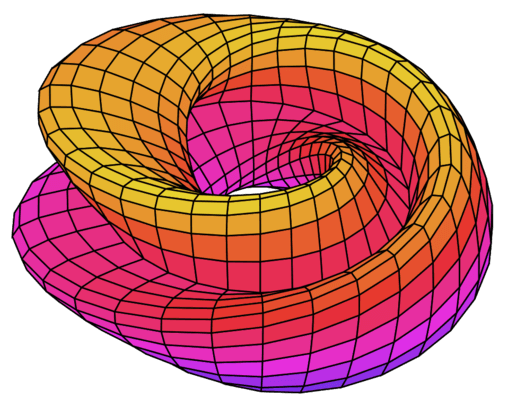How 9 Cheenta students ranked in top 100 in ISI and CMI Entrances?

# ISI & CMI Entrance Program

An outstanding program for the B.Stat and B.Math Entrances of Indian Statistical Institute and B.Sc. Math Program for Chennai Mathematical Institute.## Taught by Masters

The college mathematics program at Cheenta is created by researchers from leading universities in India and abroad.

### Weekly Concept Class

Learn a Mathematical concepts a week.

### 5-days-a-week-Problem-solving

Apply your concepts in 5-days-a-week-Problem-Solving Class.

### Cheenta Genius App

Practice daily with Genius App, doubt clearing & mock tests.

## Faculty Team

The I.S.I. and C.M.I. program at Cheenta is created by students and alumni of Indian Statistical Institute and Chennai Mathematical Institute.#### SRIJIT MUKHERJEE

Director and Faculty
Indian Statistical InstituteChennai Mathematical Institute#### AR Sricharan

Faculty and Cheenta Alumni
Chennai Mathematical Institute.Faculty
Chennai Mathematical Institute.

## CURRICULUM

ISI and CMI Entrance Program curriculum consists of advanced high school mathematics and additional topics such as geometry, number theory and combinatorics.

#### NUMBER THEORY

Book: Elementary Number Theory by David Burton
Arithmetic of Remainder, Theory of Congruence, Divisibility, Theorems of Fermat, Euler, Wilson, Pythagorean Triples, Euclidean Algorithm.

#### GEOMETRY

Book: Challenges and Thrills of Pre College Mathematics by Venkatchala et al
Geometry of straight lines, triangles and circles. Advanced topics such as geometric transformations, complex numbers in geometry, mass point geometry, coordinate Geometry and trigonometry.

#### COMBINATORICS

Book: Principles and Techniques in Combinatorics by Chuang Chong Chen and Koh Khee Meng
Bijection Principle, Principle of Inclusion and Exclusion, Recurrence Relation, Pigeon Hole Principle, Permutation and Combination, Combinatorial Identitites.

#### ALGEBRA

AM - GM and Cauchy Schwarz Inequality, Complex Numbers, Theory of Equation, Functional Equation, Sequences and Series.

Book: Single Variable Calculus by Maron
Real numbers, Limit, Continuity, Differential Calculus, Rolle's Theorem, Integral Calculus, Fundamental Theorem of Calculus, Graphing Techniques.

# What our students say...

• Our experience with Cheenta has been excellent. Even though my son started in Middle School, they understood his Math level and took personal interest in developing a long term plan considering his strengths and weakness areas.• We think Cheenta is rendering an excellent service to humanity by identifying young mathematical minds and nurturing them towards becoming inspired mathematicians of the future.#### Jayanta Majumdar (Glasgow, UK)

• My fear of mathematics has completely gone away. And I have started to believe in myself more. They have helped me in every problem. Thank you Cheenta.# Knowledge Partner